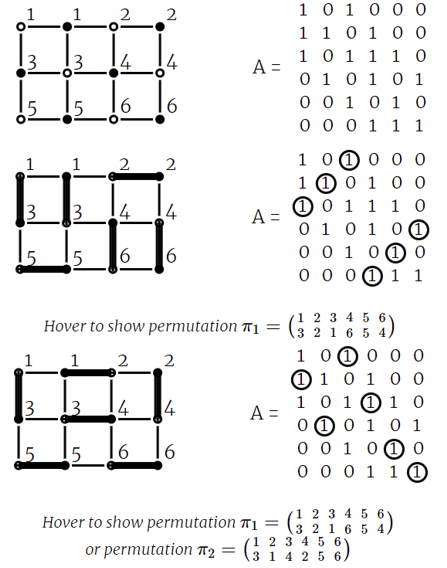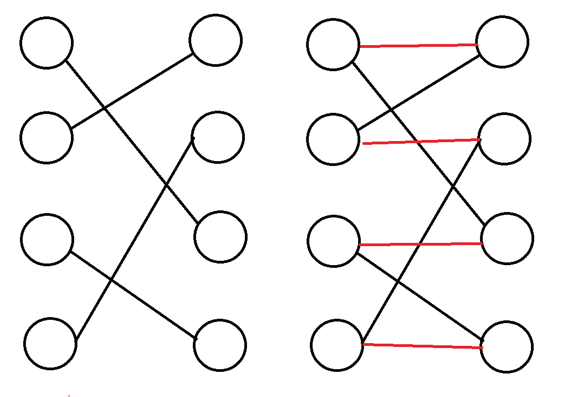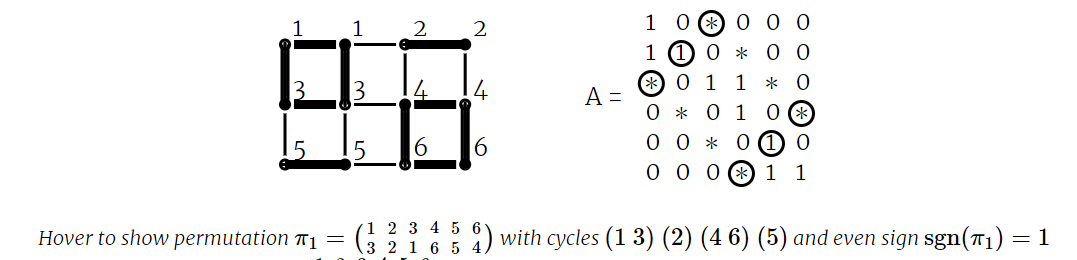#### 二分图完美匹配计数#### 网格图完美匹配计数• $e_H$ 水平边，同时存在于完美匹配中。
• $e_B$ 水平边，但是不存在于完美匹配中，也就是加入的连接左右对应点的红边，也是上图中 $(1,1),(4,4)$ 这种边。
• $e_V$ 竖直边，它一定在完美匹配中。

#### 优化高斯消元• $e_B$ 表示环里被选中的边的数量；
• $e_H$ 表示环里完美匹配中的横边数量；
• $e_V$ 表示环里竖直边的数量。